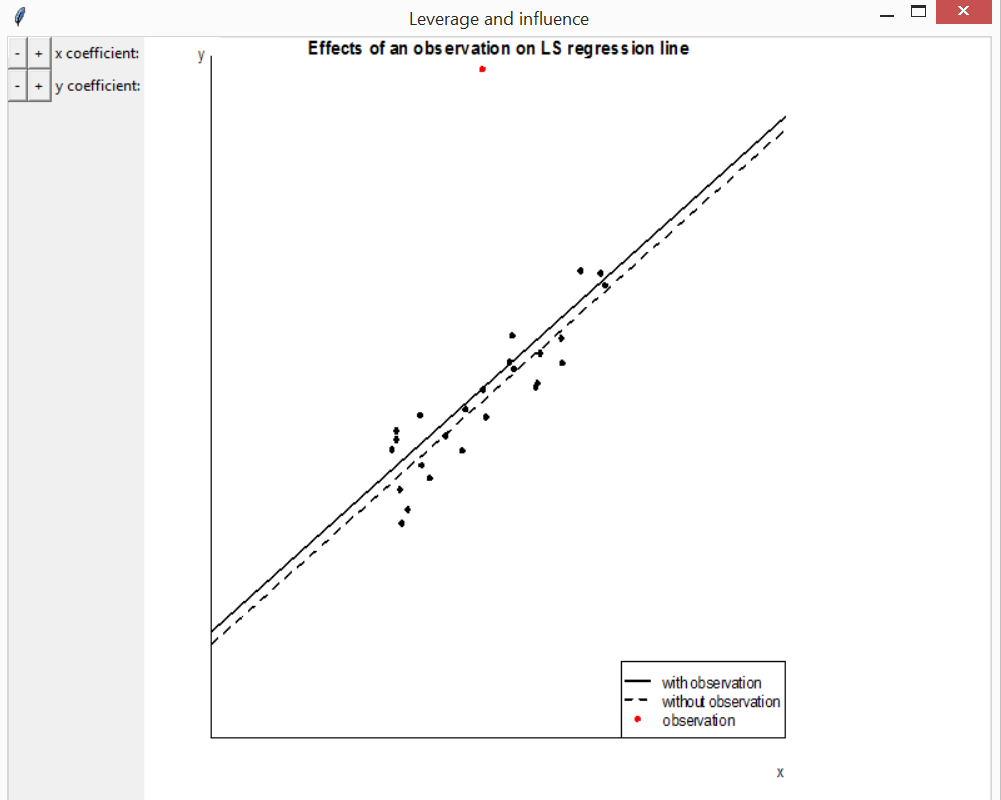# An overview of smovie

#### 2021-10-31

The smovie package provides movies to help students to understand statistical concepts. The rpanel package (Bowman et al. 2007) is used to create interactive plots that move in response to changes in parameter values. Buttons that can be held down in order to animate the plots. This vignette provides an overview of the movies that are available currently, using screenshots from the movies. Any comments, or suggestions for further movies, are gratefully received.

The function movies creates a panel from which examples of the movies can be launched. It is based on the function rp.cartoons in the rpanel package, and the image that appears in this panel has been taken from there. These examples have been set up with sensible default arguments.

library(smovie)
#> Package rpanel', version 1.1-5: type help(rpanel) for summary information
movies()

For greater control of an individual example call the relevant function directly. These functions have an argument panel_plot that controls whether the movie appears in inside the panel (TRUE) or in the standard graphics window (FALSE). The default is TRUE, partly because my experience is that holding down buttons to animate the plots works better in this case.

## Probability distributions

The functions discrete and continuous create interactive plots to illustrate how the behaviour of some common probability distributions depends on their parameter values. There are buttons to change the parameter values and to switch between displaying the p.m.f./p.d.f. and the c.d.f. These functions also allow you to provide their own distribution. If you are calling discrete or continuous directly it may be useful to use the argument var_support (for discrete) or var_range (for continuous) to fix the scale on the horizontal axis in order better to see the effects of changing the parameter values. Otherwise, for some of the distributions, the scale on the horizontal axis will react to changes in the parameters and mask the effects of any changes in location and scale parameters.

### Discrete distributions

discrete(distn = "binomial")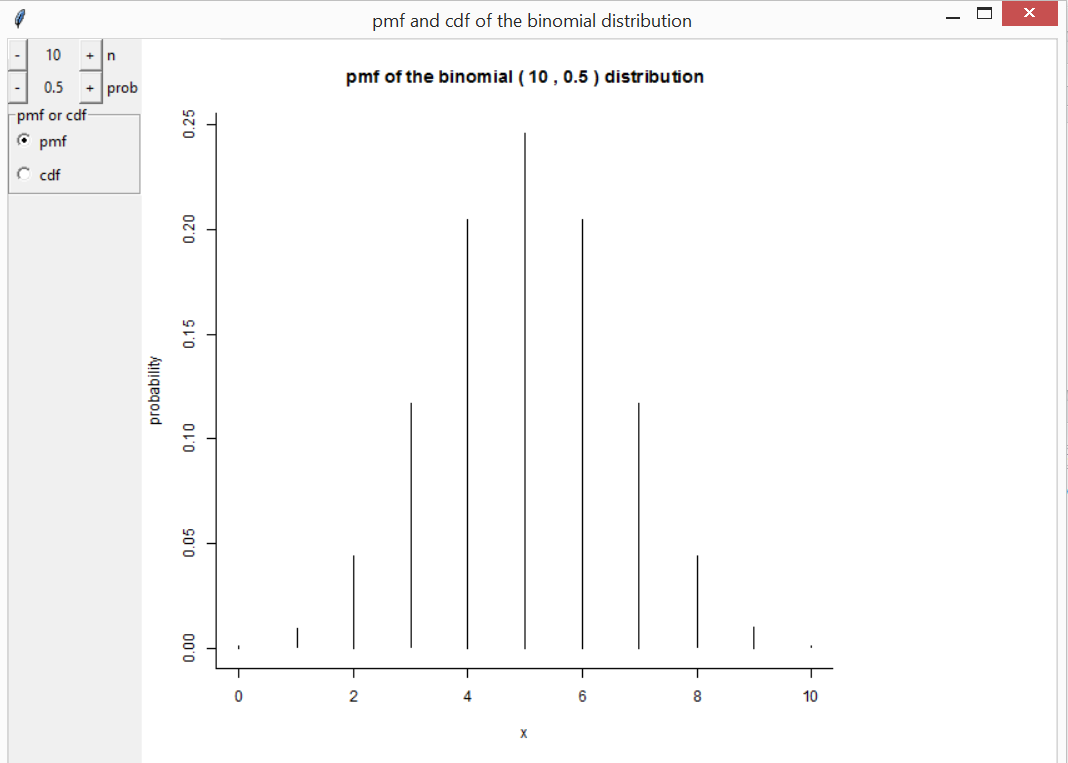### Continuous distributions

continuous(distn = "gamma")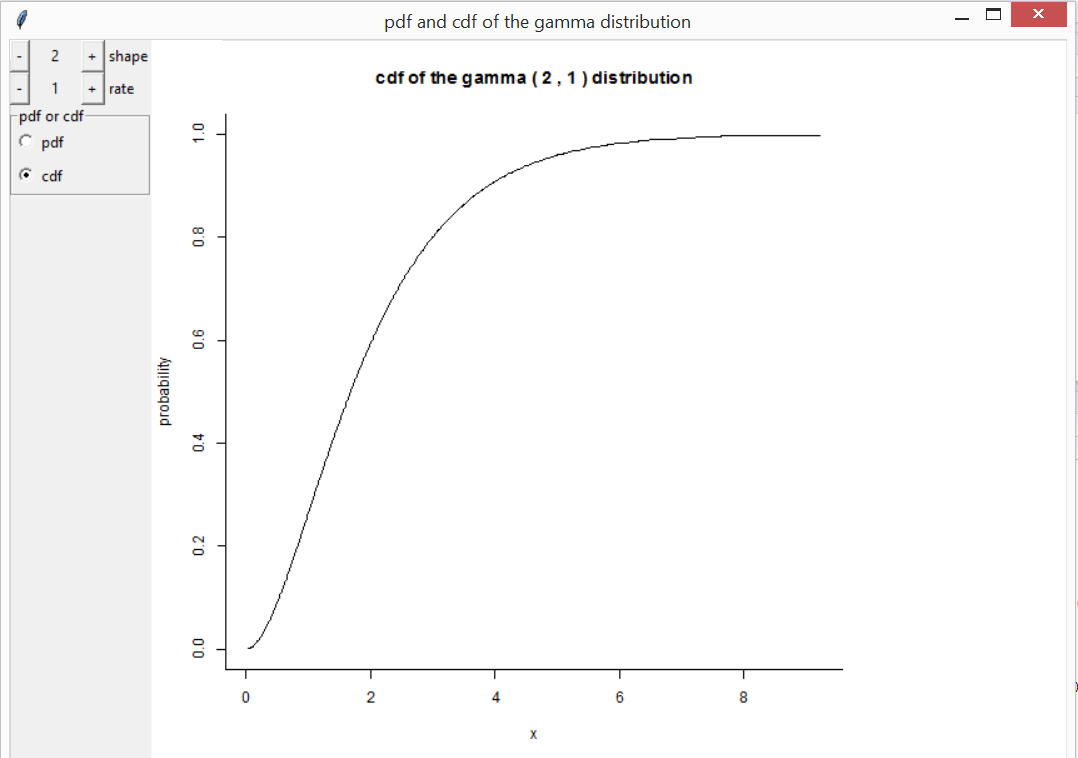## Sampling distributions

Three movies illustrate the general idea of the sampling distribution of a statistic.

### Central Limit Theorem

Consider $$n$$ independent and identically distributed random variables $$X_1, \ldots, X_n$$, each with mean $$\mu$$ and finite variance $$\sigma^2$$. Let $$\bar{X} = (1/ n) \sum_{i=1}^n X_i$$. The (classical) Central limit theorem (CLT) states that as $$n \rightarrow \infty$$ $$\sqrt{n}(\bar{X} - \mu) / \sigma$$ converges in distribution to a standard normal N(0, 1) distribution. Therefore, the mean of a sufficiently large number of such variables has approximately a N($$\mu, \sigma^2/n$$) distribution.

The function clt can be used to illustrate the convergence of the distribution of the sample mean to a normal limit. Several continuous and discrete distributions for the underlying variables are available. There are buttons to change the value of $$n$$ and to simulate another sample of size $$n$$. As the simulations progress a histogram of the sample means can be compared to the normal approximation to the sampling distribution.

clt(distn = "exponential")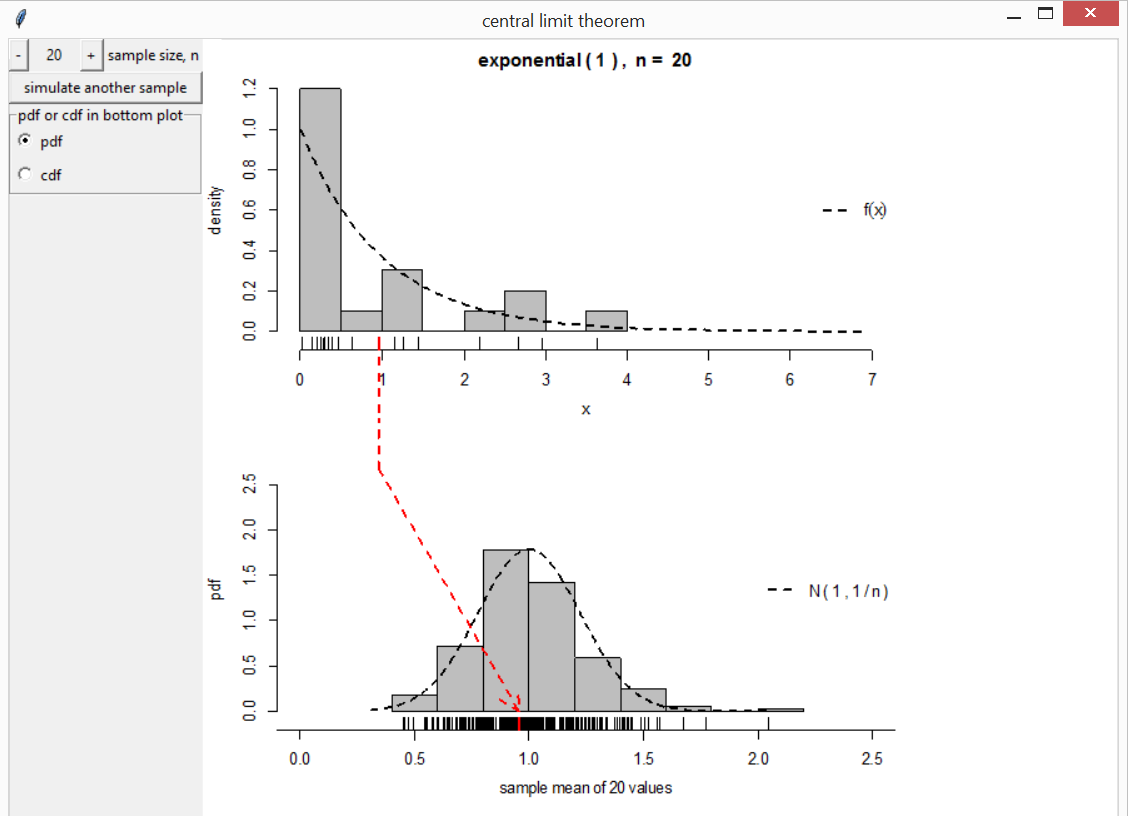clt(distn = "poisson")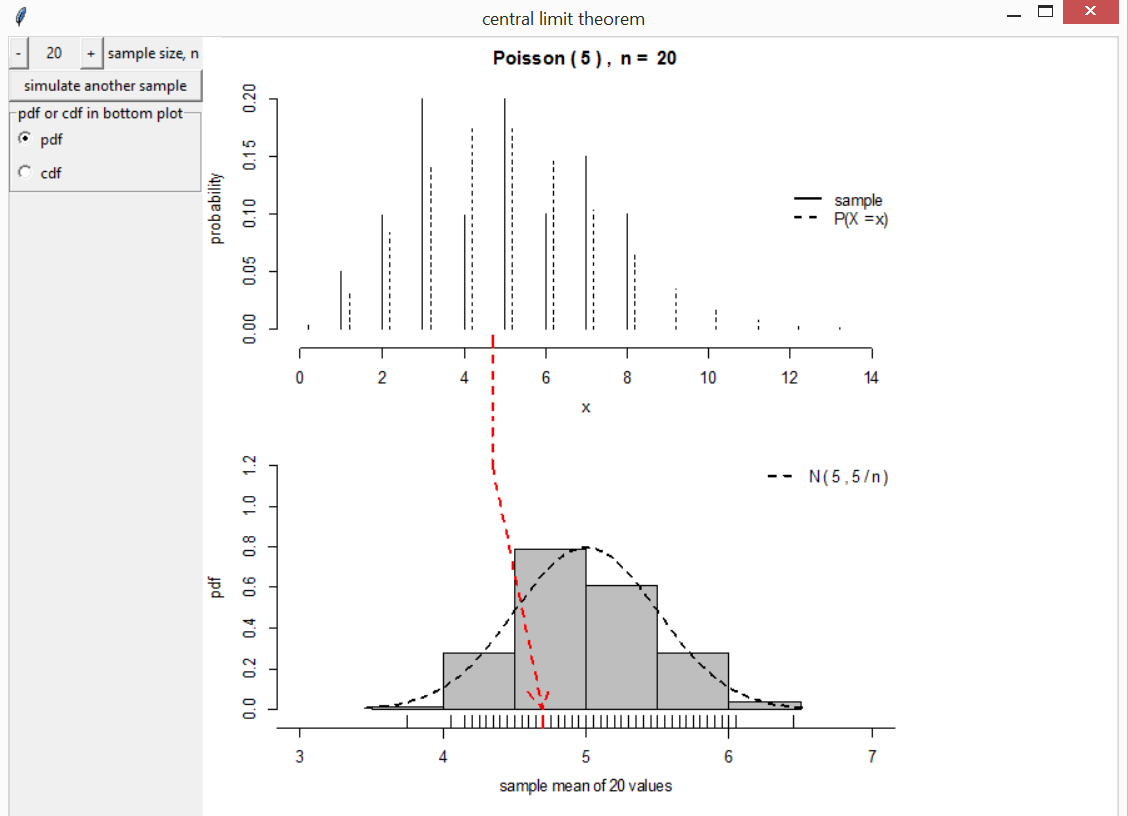### Central Limit Theorem for sample quantiles

A similar limit theorem holds for sample quantiles. Suppose that we are interested in the limiting distribution of the $$100p\%$$ sample quantile of independent and identically distributed random variables, each with probability density function $$f$$ and $$100p\%$$ quantile $$\xi(p)$$. The limiting distribution is normal with mean $$\xi(p)$$ and standard deviation $$\sqrt{p(1-p)} / n f(\xi(p))$$, provided that $$f(\xi(p))$$ is positive. See Lehman (1999) for details.

The function cltq illustrates this theorem, based here on samples from a gamma(2, 1) distribution.

cltq(distn = "gamma")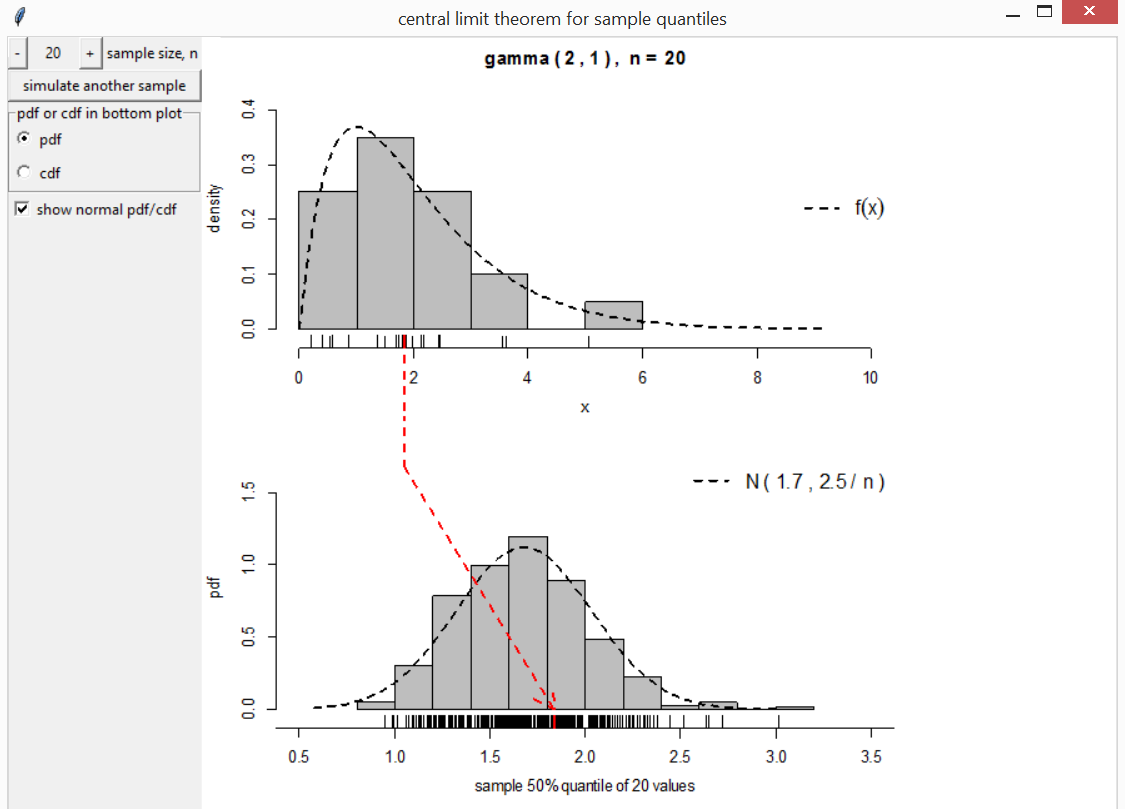### Extremal Types Theorem

The Extremal Types Theorem is rather like the CLT but applied to the sample maximum instead of the sample mean. Loosely speaking, it states that, in many situations, the maximum of a large number $$n$$ of independent random variables has approximately a Generalized Extreme Value (GEV) distribution with shape parameter $$\xi$$. See Coles (2001) for an introductory account of extreme value theory.

The function ett can be used to illustrate the convergence of the distribution of the sample maximum to a GEV limit. Several continuous distributions for the underlying variables are available corresponding to different values of the limiting shape parameter $$\xi$$. ett works in essentially the same way as clt except that the exact sampling distribution of the sample maximum is also displayed.

ett(distn = "exponential")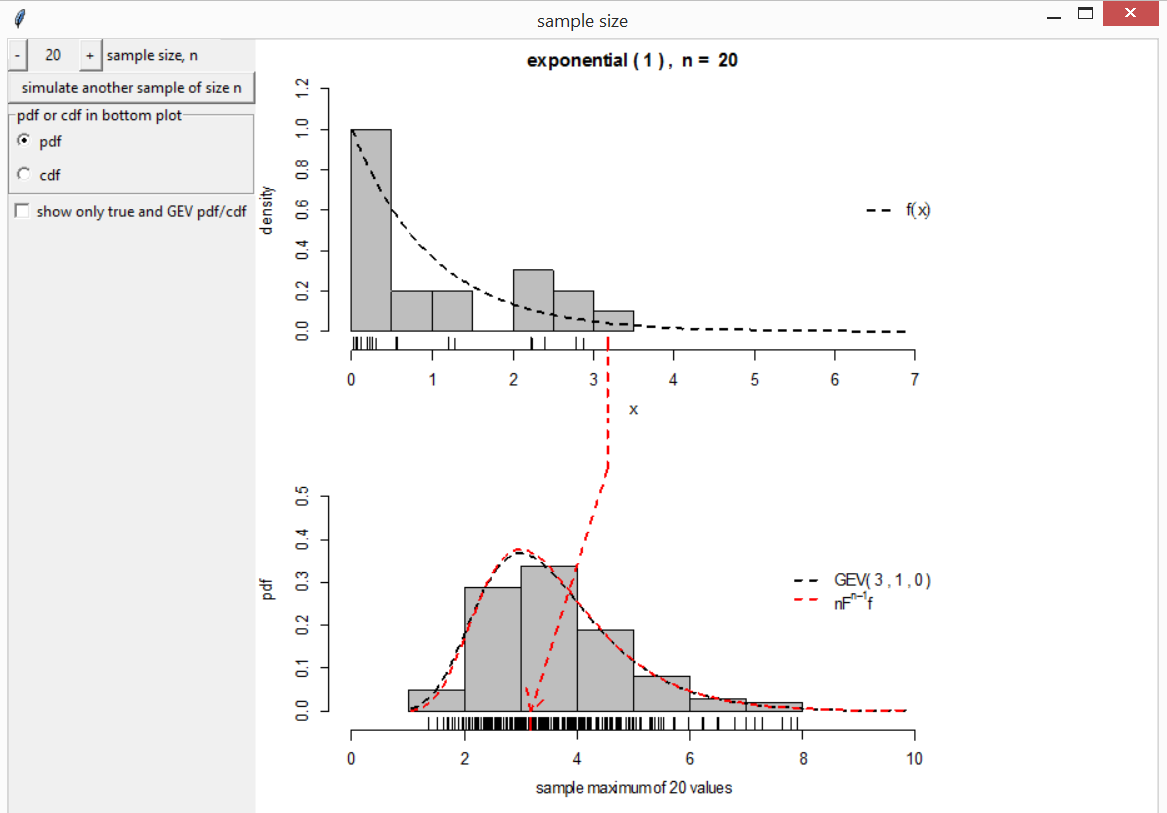### Mean vs median

The function mean_vs_median produces a movie to compare the sampling distributions of the sample mean and sample median based on a random sample of size $$n$$ from either a standard normal distribution or a standard Student’s $$t$$ distribution.

If we sample from a standard normal distribution then the sample mean has the smaller sample variance, as illustrated in the following movie.

mean_vs_median()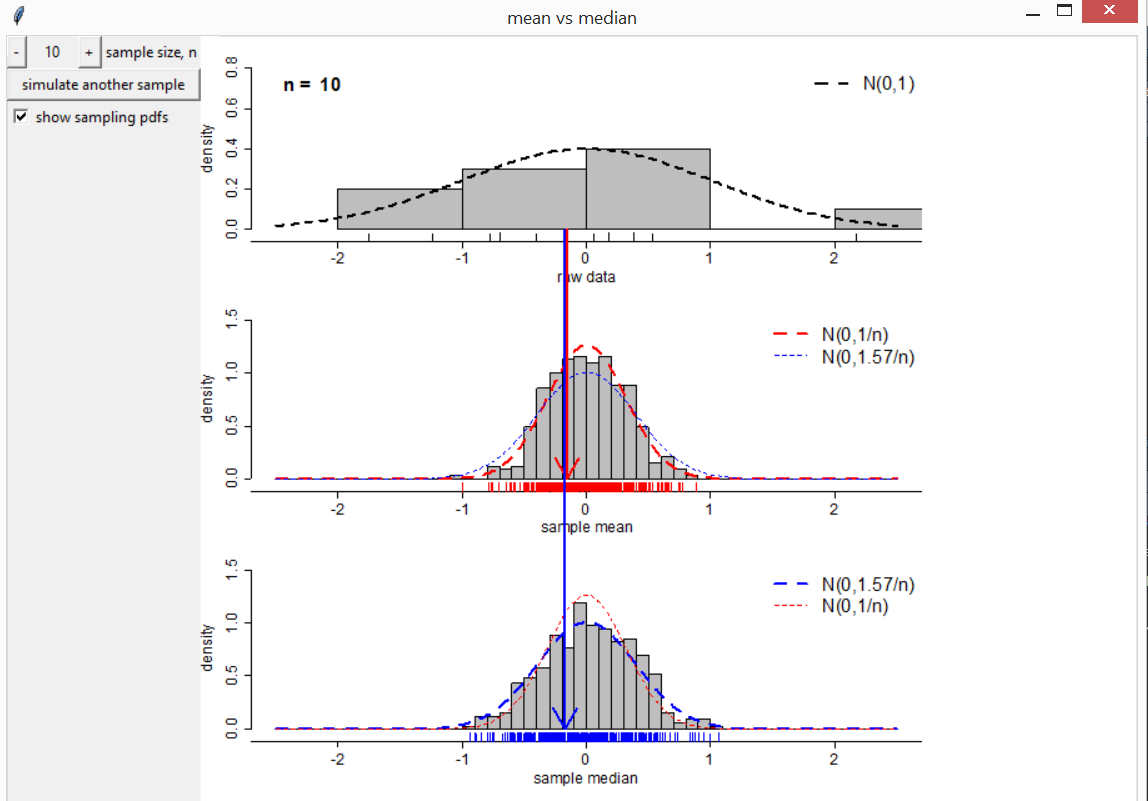If we sample from a Student’s $$t$$ distribution with 2 degrees of freedom then the next movie reveals that the sample median has the smaller sampling variance.

mean_vs_median(t_df = 2)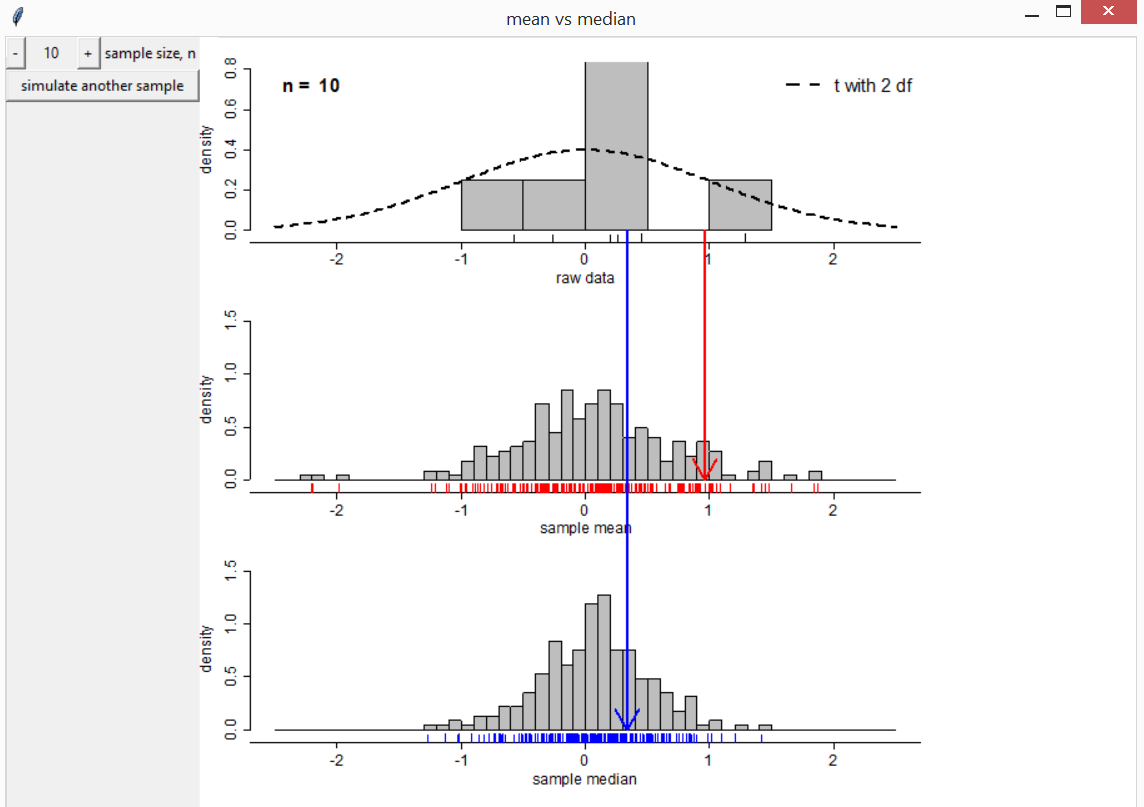### Product Moment Correlation Coefficient

The function correlation illustrates the sampling distribution of the product moment correlation coefficient, under sampling from a bivariate normal distribution. There are buttons to change the values of $$n$$ and the true correlation $$\rho$$ and to simulate another sample of size $$n$$. A histogram of the sample correlation coefficients is compared to the exact pdf of the sampling distribution. There is also an option to switch to a display of Fisher’s $$z$$-transformation $$[\ln(1+\rho) - \ln(1-\rho)] / 2$$. Then the histogram can be compared both to the exact distribution and to a normal approximation to this distribution.

correlation(rho = 0.8, n = 30)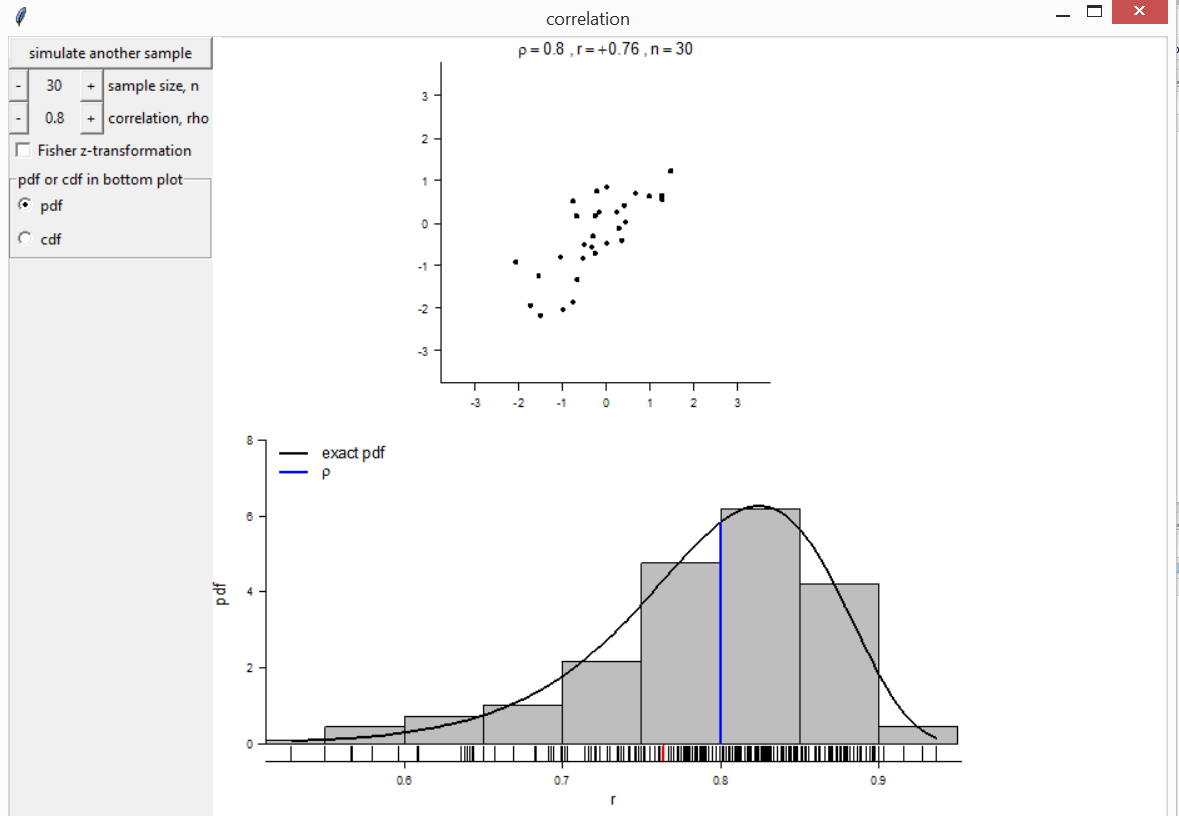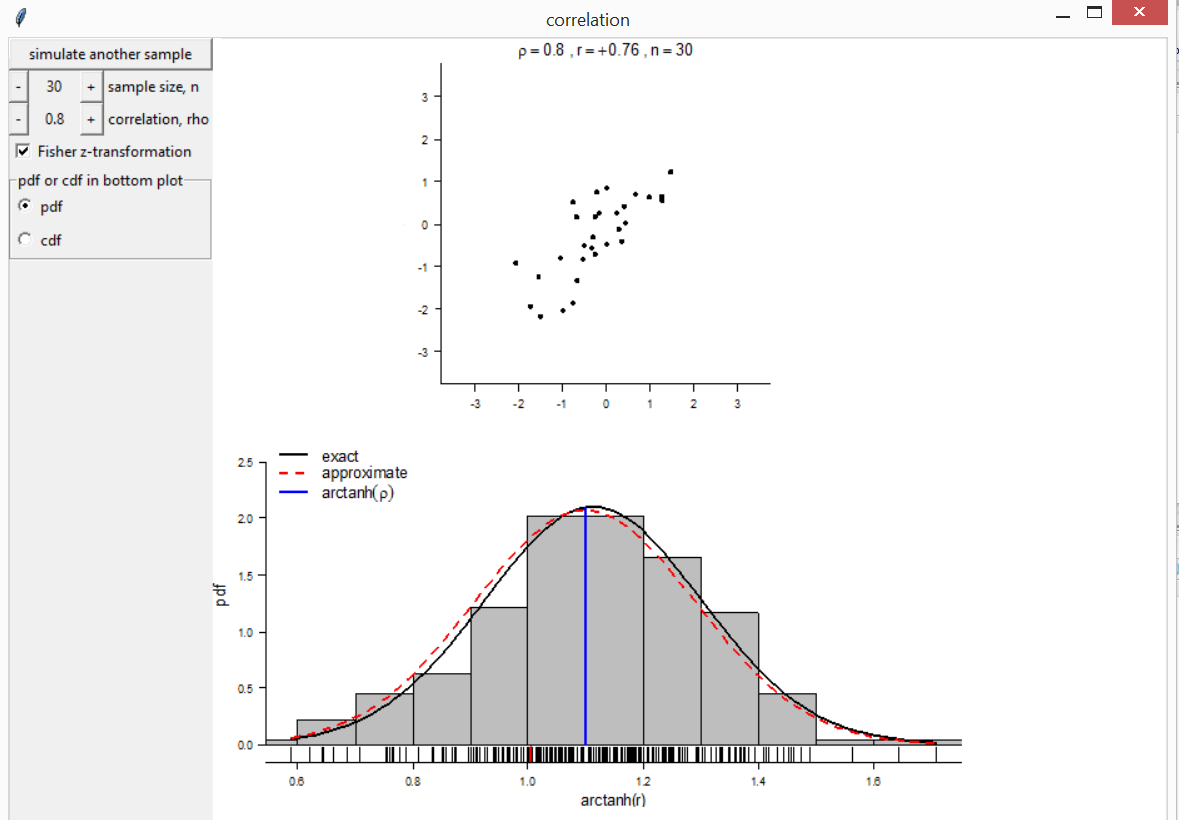## Regression

### Leverage and influence

The function lev_inf creates a plot to visualise the effect on a least squares regression line of moving a single observation, in order to illustrate the concepts of leverage and influence. In the first plot below the effect of increasing the $$y$$-coefficient of the red observation is far greater when the $$x$$-coefficient is far from the mean $$x$$-coefficient of the other observations.

lev_inf()`